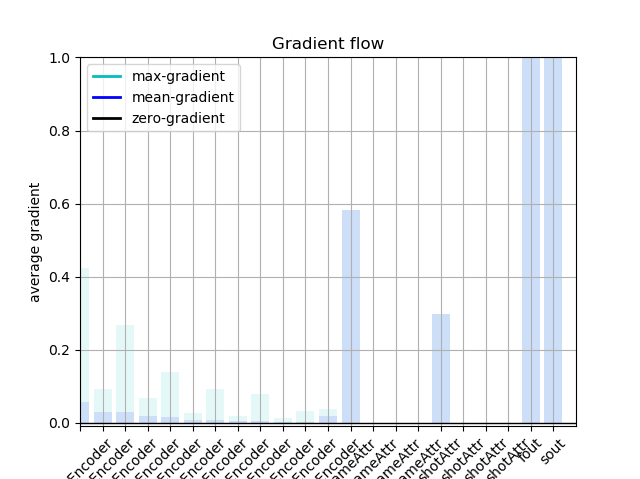# Gradient becomes 0 for 1D convolutions

I’m trying to implement the attention module with 1D convolution.
Here’s my custom attention module for that.

``````class convSelfAttention(nn.Module):
def __init__(self, in_dim):
super(convSelfAttention, self).__init__()
self.channel_in = in_dim
self.query_conv = nn.Conv1d(in_channels=in_dim, out_channels=in_dim//2, kernel_size=1)
self.key_conv = nn.Conv1d(in_channels=in_dim, out_channels=in_dim//2, kernel_size=1)
self.value_conv = nn.Conv1d(in_channels=in_dim, out_channels=in_dim, kernel_size=1)
self.gamma = nn.Parameter(torch.zeros(1))
self.activ = nn.Softmax(dim=-1)

def forward(self, x):
p_query = self.query_conv(x).permute(0, 2, 1)
p_key = self.key_conv(x)
energy = torch.bmm(p_query, p_key)
attention = self.activ(energy)
p_value = self.value_conv(x)

out = torch.bmm(p_value, attention.permute(0, 2, 1))
out = self.gamma * out + x
out = out.permute(0, 2, 1)
return out
``````

However, the gradients are 0 except ‘self.gamma’.
For your convenience, I plot the gradient flow of my entire architecture.(The last two layers are fc and all layers from ‘~~Encoder’ are convolutions)